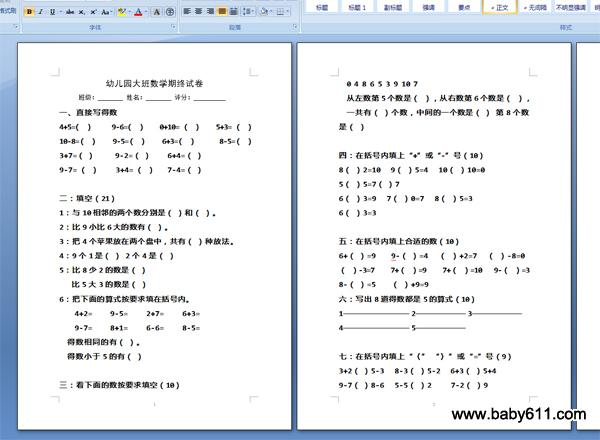幼儿园大班数学期终试卷幼儿园大班数学期终试卷

班级：         姓名：         评分：

一、直接写得数

4+5=（ ）     9-6=（ ）    0+10= （ ）    5+3= （ ）

10-8=（ ）    9-5=（ ）    6+3=（ ）      8-5=（ ）

3+7=（ ）     9-2=（ ）    6+4=（ ）

9-7= （ ）    3+4= （ ）   7-4=（ ）

二：填空（21）

1：与10相邻的两个数分别是（ ）和（ ）。

2：比9小比6大的数有（ ）。

3：把4个苹果放在两个盘中，共有（ ）种放法。

4：9个1是（ ） 2个4是（ ）

5：比8少2的数是（ ）

比5大3的数是（ ）

6：把下面的算式按要求填在括号内。

4+2=     9-5=     2+7=     6+3=

9-7=     8+1=     6-6=     8-5=

得数相同的有（ ）。

得数小于5的有（ ）

三：看下面的数按要求填空（10）

0 4 8 6 5 3 9 10 7

从左数第5个数是（ ），从右数第6个数是（ ），

一共有（ ）个数，中间的一个数是（ ） 第8个数是（ ）

四：在括号内填上"+"或"-"号（10）

8（ ）2=10   9（ ）5=4   10（ ）10=0

5（ ）5=7（ ）7

6（ ）3=9   7（ ）0=7   8（ ）5=3

6（ ）3=3

五：在括号内填上合适的数（10）

6+（ ）=9    9-（ ）=4   （ ）+2=7   （ ）-8=0

（ ）-3=7    7+（ ）=9    7+（ ）=10   9-（ ）=3

8-（ ）=5    （ ）+9=9

六：写出8道得数都是5的算式（10）

1-------- 2------ 3------

4-------- 5------

七：在括号内填上"〈" "〉"或"="号（9）

3+2（ ）5-3   8-3（ ）5-2   6+3（ ）5+4

9-7（ ）8-6   5-5（ ）2     7-2（ ）9

5+4（ ）3+2   0+9（ ）9     5+3（ ）9

八：解决问题（10）

1：小红有4朵花，小明有3朵花，小刚有2朵花，三人一共有多少朵花？

2：小华家有4口人，小刚家比小华家多2口人，小刚家有几口人？

• 幼儿园试卷分类
• 将此试卷分享到：
• 大班试卷最新更新